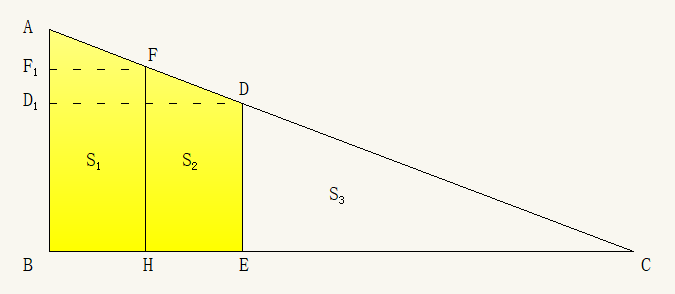Questions in category: 初等几何 (Elementary Geometry)

## 1. 设 $\triangle ABC$ 中 $\angle CAB=60^{\circ}$, $AD$ 是 $\angle CAB$ 的角平分线. 并且 $|AC|=1$, $|AB|=3$. 试求 $|AD|$ 的长.

Posted by haifeng on 2019-01-17 22:47:25 last update 2019-01-17 22:48:43 | Answers (0) | 收藏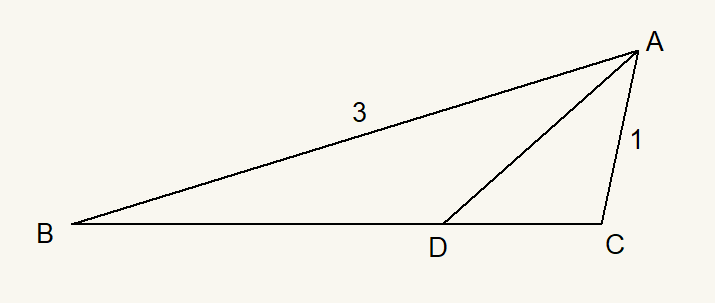[hint] 参考问题2191

## 2. 将直角三角形 $\triangle EAB, \triangle BFC, \triangle CDA$ 如下图放置, 其中 $\angle EAB=\angle BFC=\angle CDA=90^{\circ}$

Posted by haifeng on 2019-01-17 22:03:48 last update 2019-01-17 22:20:20 | Answers (0) | 收藏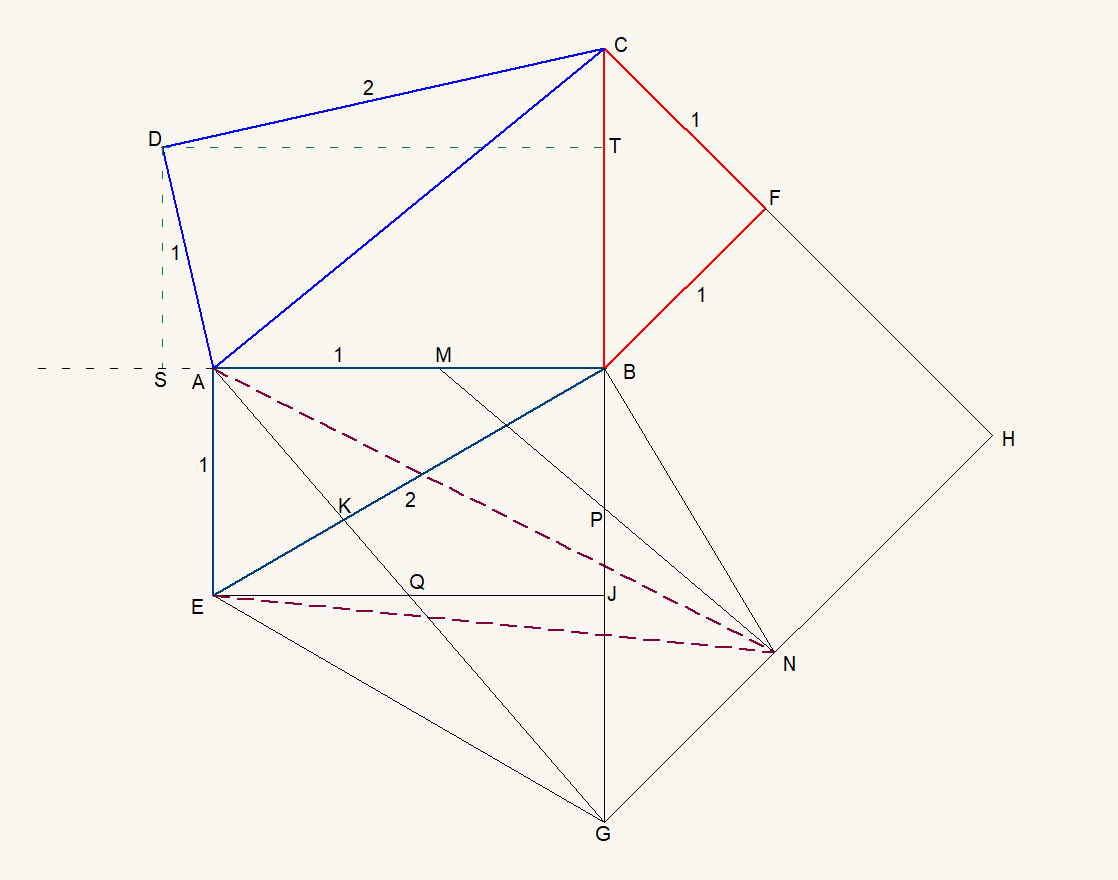• 设 $|AE|=|BF|=|CF|=|DA|=1$,  $|EB|=|CD|=2$.
• 延长 $CB$ 至 $G$, 使得 $|BG|=2$;
• 延长 $CF$ 至 $H$, 使得 $GH\perp CH$.
• 连接 $EG$, $AG$.
• 过点 $B$ 作 $BN\perp EB$, 且 $BN$ 交 $GH$ 于 $N$.
• 在 $AB$ 上取点 $M$, 使得 $|AM|=1$. 连接 $MN$.
• $EJ$ 平行于 $AB$, 且 $EJ$ 交 $AG$ 于点 $Q$.
• 过点 $D$ 作 $DT\perp BC$ 于 $T$, 作 $DS\perp BA$ 延长线 于 $S$.

(1) $\triangle AEG$ 与 $\triangle MBN$ 相似.

(2) $\frac{|AN|}{|EN|}=\frac{\sqrt{5}}{2}$.

(3) $|DT|=2|DS|$.

## 3. [Langley's problem] Triangle 20 80 80

Posted by haifeng on 2018-03-23 14:27:21 last update 2018-03-24 00:36:00 | Answers (2) | 收藏

Langley 问题是一道比较有名的初等几何题目, 有很多证明方法或求解方法. (https://www.cut-the-knot.org/triangle/80-80-20/IndexToClassical.shtml 上给出了12种方法.)

http://www.arbelos.co.uk/Papers/Triangle-problem.pdf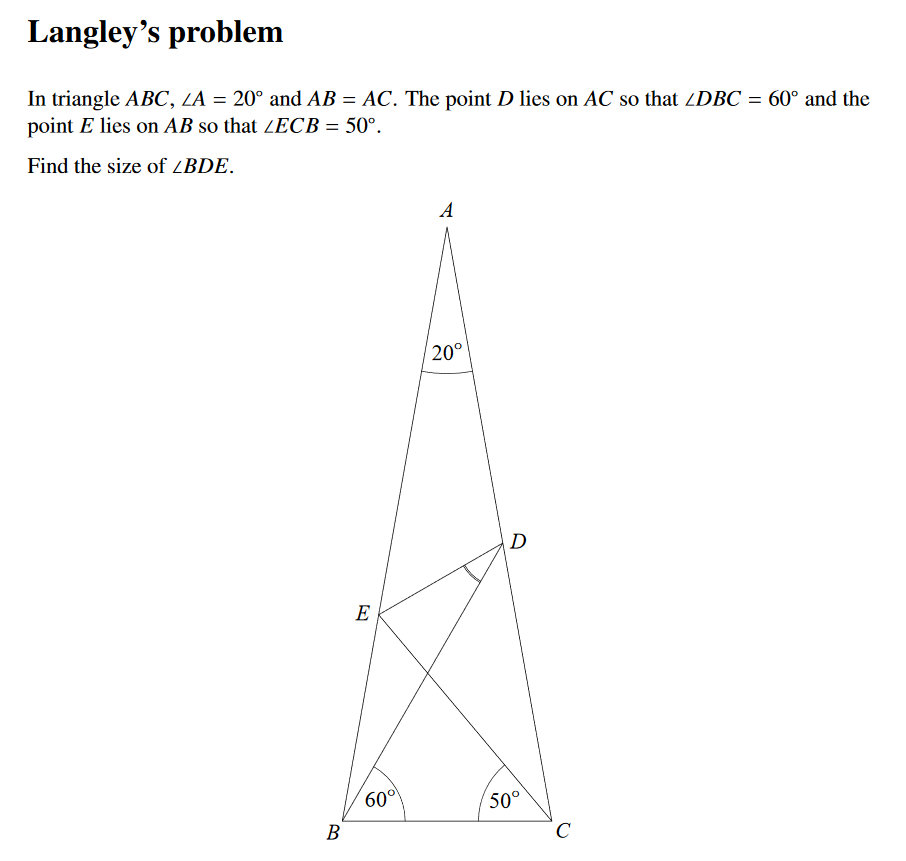Remark:

References:

https://www.cut-the-knot.org/triangle/80-80-20/index.shtml

## 4. 证明图中两块阴影部分的面积相等, 即 $A=B$.

Posted by haifeng on 2018-03-11 16:57:15 last update 2018-03-23 14:24:24 | Answers (1) | 收藏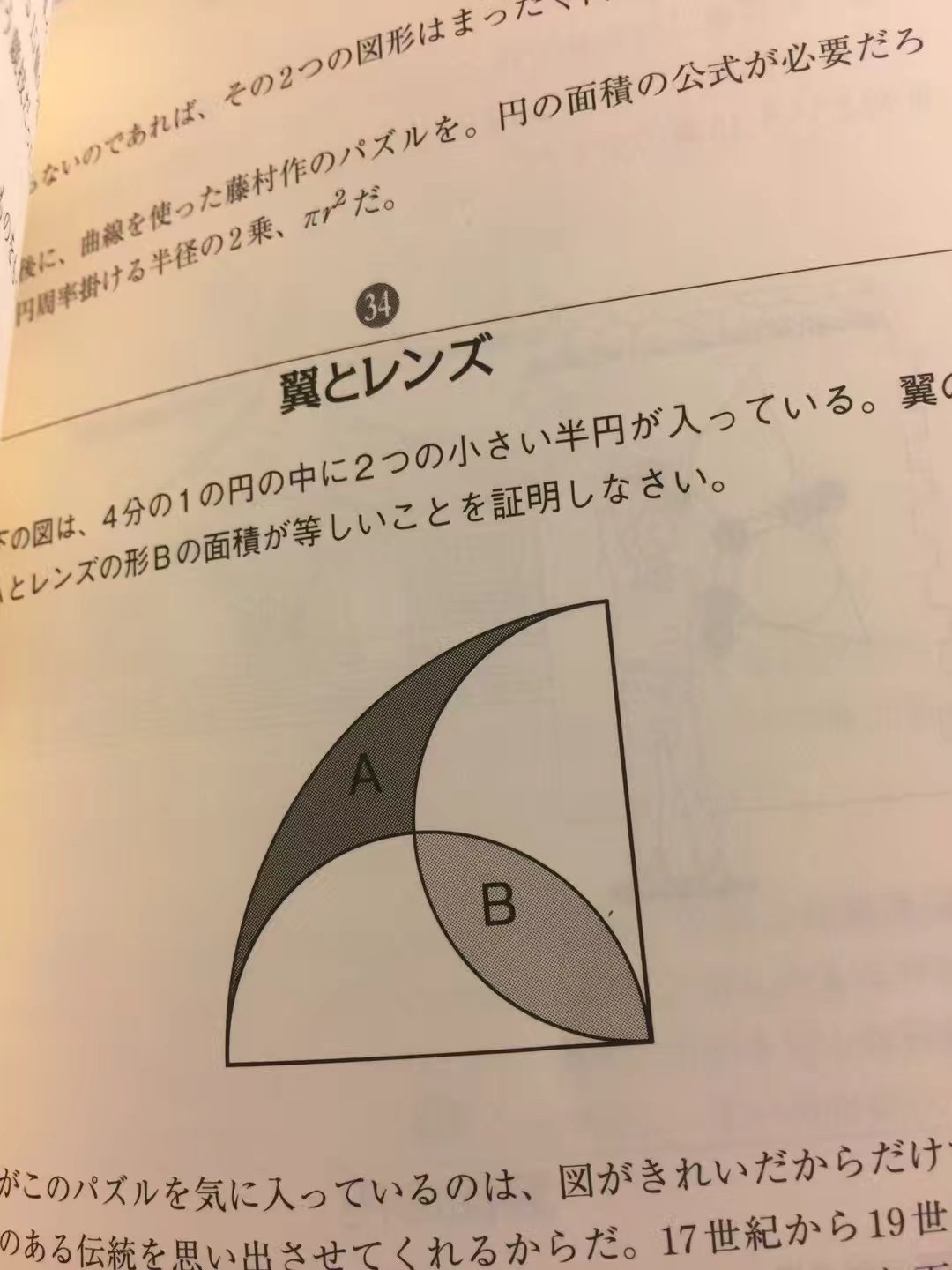The picture is provided by Lei LIU.

La photo est fournie par Lei LIU.

## 5. 直角三角形中由两条平行于某一直角边的直线(这三条直线的间隔相等)构成了两个梯形, 它们的面积决定了另一个三角形的面积.

Posted by haifeng on 2017-10-19 16:28:08 last update 2017-10-20 20:29:13 | Answers (1) | 收藏